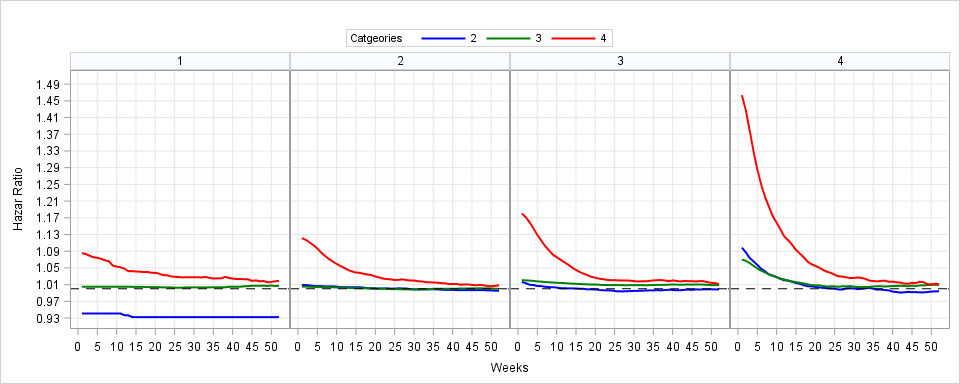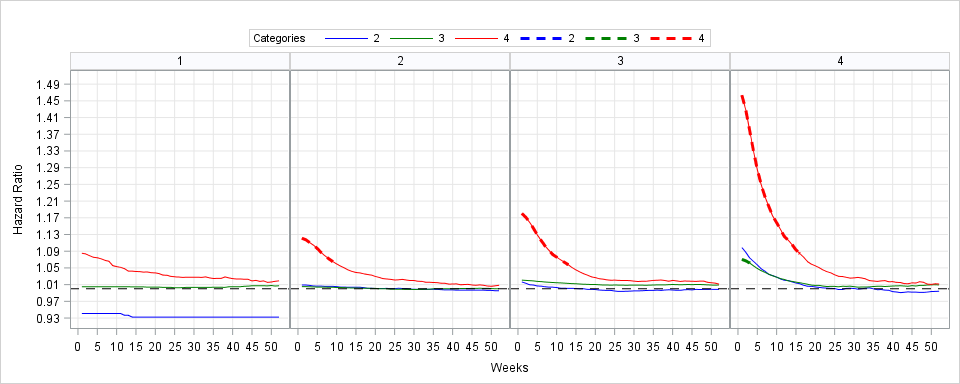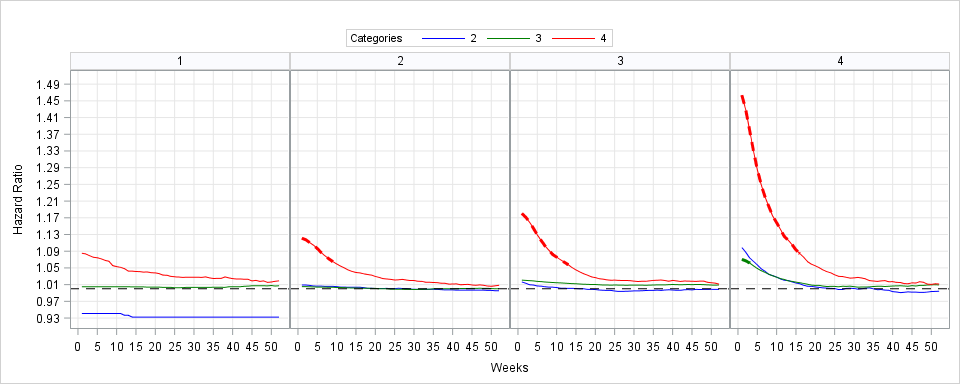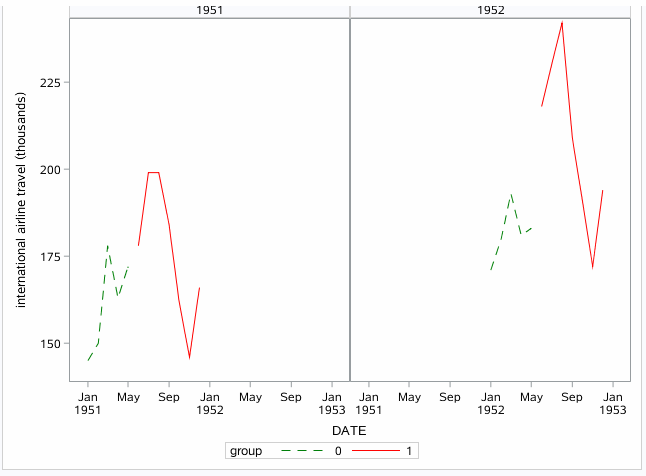## Highlight the part of the lines when corresponding p-values are statistically signifiant

Hi Folks:

I'd like to highlight the part of the lines when corresponding p-values are statistically signifiant. Desired graph would have the line parts that are associated with 95% CI not inclusive of 1 bolded or bolded/dashed in a way that is more prominent than the line parts that are not significant.

Any technical possibilities there and know how?

Data attached.``````
ods graphics/width=10in height=4in;
proc sgpanel data=p.PAR_PANC_FU;
panelby agegrp/ novarname columns=4 rows=1;
styleattrs DATACONTRASTCOLORS=(BLUE green red);
series  x=FU y=HazardRatio/group=stage lineattrs=(pattern=solid) LEGENDLABEL='HAZARD RATIO' lineattrs=(thickness=2);
keylegend/ title="Catgeories" position=TOP;
REFLINE 1 / LABEL = (' ') lineattrs=(color=black pattern=dash thickness=1.5);
colaxis label='Weeks' min=1 max=100 grid values=(0 to 52 by 1);
rowaxis label='Hazar Ratio'
fitpolicy=thin grid  values=(0.93 to 1.5 by 0.01);
/*INSET N PROBF/position=TOPRIGHT TEXTATTRS=(Color=BLUE Family=Arial Size=10)*/
title " ";
run;

data sig; set Par_panc_fu;
length one \$8;
if HRLowerCL>1 and HRUpperCL>1 then one='over'; else
if HRLowerCL<1 and HRUpperCL<1 then one='under'; else
one='non_sig';
run;

proc print data=sig;
where one ne 'non_sig';
run; ``````

1 ACCEPTED SOLUTION

Accepted Solutions

## Re: Highlight the part of the lines when corresponding p-values are statistically signifiant

I think it's something you can do yourself. And I can't download attachments so can't work with data or anything.

1. Create a new variable with only significant values notes (IF/THEN statement most likely)

2. Add a new series statement, referring to the new variable and specifying a thicker line. Not exactly sure how that will work with SGPANEL but should be fine.

It's one approach, there are likely others.

10 REPLIES 10

## Re: Highlight the part of the lines when corresponding p-values are statistically signifiant

Add an overlay of a band or a thicker line for parts that are significant.
Create a new variable that is the same as the graphed values but only present when the data is significant. Then graph that series using a bolder line structure or band so that it shows up more on the graph. It'll be overlaid so no issues otherwise.

## Re: Highlight the part of the lines when corresponding p-values are statistically signifiant

Reeza, do you have time to show me in code?

## Re: Highlight the part of the lines when corresponding p-values are statistically signifiant

I think it's something you can do yourself. And I can't download attachments so can't work with data or anything.

1. Create a new variable with only significant values notes (IF/THEN statement most likely)

2. Add a new series statement, referring to the new variable and specifying a thicker line. Not exactly sure how that will work with SGPANEL but should be fine.

It's one approach, there are likely others.

## Re: Highlight the part of the lines when corresponding p-values are statistically signifiant

Worked as shown below. Thank you!!!``````

ods graphics/width=10in height=4in;
proc sgpanel data=p.sig;
panelby agegrp/ novarname columns=4 rows=1;
styleattrs DATACONTRASTCOLORS=(BLUE green red);
series  x=FU y=HazardRatio/group=stage lineattrs=(pattern=solid) LEGENDLABEL='HAZARD RATIO' lineattrs=(thickness=1);
series  x=FU y=one/group=stage lineattrs=(pattern=dash) lineattrs=(thickness=3);
keylegend/ title="Categories" position=TOP;
REFLINE 1 / LABEL = (' ') lineattrs=(color=black pattern=dash thickness=1.5);
colaxis label='Weeks' min=1 max=100 grid values=(0 to 52 by 1);
rowaxis label='Hazard Ratio'
fitpolicy=thin grid  values=(0.93 to 1.5 by 0.01);
INSET N PROBF/position=TOPRIGHT TEXTATTRS=(Color=BLUE Family=Arial Size=10)
BORDER;
title " ";
/*format stage stage. agegrp agegrp.; */
run;
``````

## Re: Highlight the part of the lines when corresponding p-values are statistically signifiant

Any idea how to get rid of the legend for the dashed lines ?DanH_sas
SAS Super FREQ

## Re: Highlight the part of the lines when corresponding p-values are statistically signifiant

Put a NAME= on the SERIES you want in the legend (e.g. NAME="mySeries"), and add the following statement to the proc:

keylegend "mySeries";

If you want a TITLE on it:

keylegend "mySeries" / title="My Group Title";

Hope that helps!

Dan

## Re: Highlight the part of the lines when corresponding p-values are statistically signifiant

Great. Solved the problem.and the final code is following:

``````ods graphics/width=10in height=4in;
proc sgpanel data=p.sig;
panelby agegrp / novarname columns=4 rows=1 ;
styleattrs DATACONTRASTCOLORS=(BLUE green red);

series  x=FU y=HazardRatio/group=stage lineattrs=(pattern=solid) LEGENDLABEL='HAZARD RATIO' lineattrs=(thickness=1)
name="mySeries";

series  x=FU y=one/group=stage lineattrs=(pattern=dash) lineattrs=(thickness=3);

keylegend "mySeries"/ title="Categories" position=TOP;

REFLINE 1 / LABEL = (' ') lineattrs=(color=black pattern=dash thickness=1.5);
colaxis label='Weeks ' min=1 max=100 grid values=(0 to 52 by 1);
rowaxis label='Hazard Ratio'
fitpolicy=thin grid  values=(0.93 to 1.5 by 0.01);
INSET N PROBF/position=TOPRIGHT TEXTATTRS=(Color=BLUE Family=Arial Size=10)
BORDER;
title " ";
/*format stage stage. agegrp agegrp.; */
run;``````Ksharp
Super User

## Re: Highlight the part of the lines when corresponding p-values are statistically signifiant

``````data air;
set sashelp.air;
year=year(date);
group=(month(date)>5);
if year in (1951 1952);
run;

proc print;run;

ods graphics/attrpriority=none;
proc sgpanel data=air;
panelby year/rows=1 novarname;
styleattrs datacontrastcolors=(green red) datalinepatterns=(dash solid) ;
series x=date y=air/group=group;
run;``````## Re: Highlight the part of the lines when corresponding p-values are statistically signifiant

The OP's graph already includes a GROUP statement which means more data manipulation is required to handle that 😞

## Re: Highlight the part of the lines when corresponding p-values are statistically signifiant

@Cruise wrote:

Any idea how to get rid of the legend for the dashed lines ?